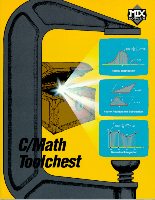# Turn your C/C++ compiler into a powerful scientific programming tool...Now you can develop sophisticated scientific and engineering applications using your favorite C or C++ compiler. With a broad range of mathematical functions, the C/Math Toolchest (TM) gives C as much number crunching power as FORTRAN. More than 135 functions provide support for:

• Probability and Statistics:
including uniform, normal, binomial, Poisson, and hypergeometric distributions, and least-squares regression.
• Complex Arithmetic and Linear Algebra:
including a comprehensive set of vector and matrix operations, and solutions to simultaneous linear equations.
• Numerical Analysis and Digital Signal Processing:
including integration, differentiation, interpolation, root finding, convolution, FFTs, power spectrum analysis, and filter design.

To graphically view the results of your number crunching, we've also included GRAFIX (TM), a graphical data analysis program. The GRAFIX (TM) program makes it easy to edit, plot, interpolate, or perform regression analysis of your data.

You may also purchase the C source code for the library and GRAFIX (TM) program. The C/Math Library Source works with any ANSI standard C or C++ compiler. The C/Math GRAFIX Source works with the C and C++ compilers from MIX (R), Borland (R), and Microsoft (R). Prebuilt libraries for the DOS versions of these compilers are included with the C/Math Toolchest.

Prices:

• C/Math Toolchest \$29.95
• C/Math Library Source \$10.00
• C/Math GRAFIX Source \$10.00

Not Copy Protected * Royalty Free
30-Day Money Back GuaranteeFunction descriptionsProduct List